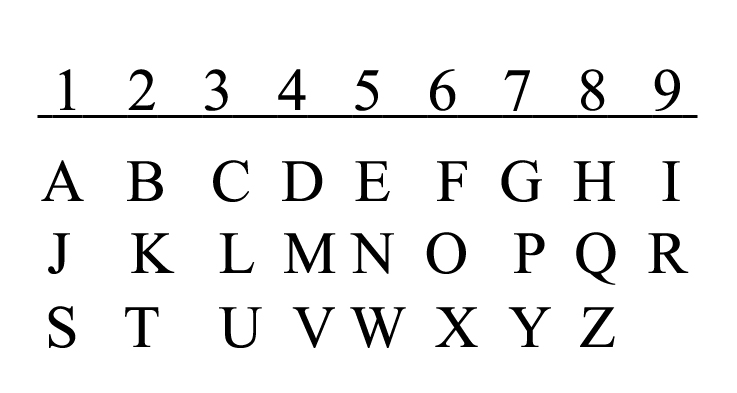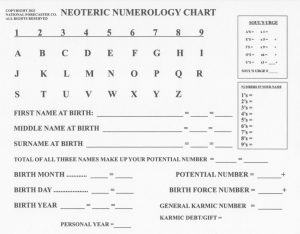# Numerology

Numerology can be traced back to the mathematician Pythagoras of Samos, Ionia who lived from 569 BC to 475 BC. Pythagoras was interested in the principles of mathematics, the concept of number, the concept of a triangle and other mathematical figures. He was also a teacher of Metaphysics. Unfortunately, due to his insistence on secrecy, his metaphysical teachings have been lost to time.

Pythagoras is credited with the creation of Numerology. He was the first to talk about Vibrational Influence as it applied to numbers. He theorized that the vibration of a number was unique unto itself and that it influenced us when we vibrated around it and other numbers for long periods of time. He did not, however, invent the Numerology System. The Chinese developed his Vibrational Theory, determined the influence of each simple number and applied that to our birth names and our birth dates. We vibrate around those two numbers every second of our lives.

Juno Jordan, a Chinese native, introduced the Western World to Numerology in 1961. And, for the next 25 years until her death in 1986, a select group of Western Numerologist’s met with her each year in an effort to improve and expand Western Numerology.

In keeping with Juno Jordan’s tradition John Scarano has updated the Pythagorean System of Numerology. His updated version is known as Neoteric Numerology. Your chart will be calculated using the Neoteric Numerology System.

Calculating the Numerology Chart:

Calculation #1:

The letters in the English alphabet are converted into numbers in the following manner:

(Click Image to Enlarge)Here you simply add up all of the corresponding letter numbers from your names at birth. The letter value is determined by the number under which it falls in the above chart. The sum of these numbers will be a compound number like 53, for example. Numerology deals only with the simple numbers from 1 to 9. So, we have to add the numbers in the total sum to get the simple number from your names at birth. Thus, 53 = 5 + 3 = 8. This number is known as your Destiny Number. That is a misnomer of sorts. It probably should have been called your Potential Number. This number indicates your potential in this lifetime. John expands greatly on this number’s influence on you in your chart interpretation.

Calculation #2:

This calculation is made by converting your birth month into a number and adding it to the rest of your birthdate. For example; November 12, 1958 = 1 + 1 + 2 + 1 + 9 + 5 + 8 = 27 and 2 + 7 = 9. This number is known as your Birth Force. This number indicates the talents, skills and attitudes that you were given. This number “forces” your Destiny Number towards its potential. Again, John expands greatly on this number’s influence on you in your chart interpretation.

Other Useful Calculations:

John makes 5 more calculations from your chart. Here is the full chart that John personally developed and will use exclusively to calculate your Personal or Business Numerology Chart:

(Click Image to Enlarge)Software Used to Calculate Your Chart:

John uses no software to produce your chart. Everything is done by hand. This is important because John needs to see every number that appears in your chart. Numerology programs give you the sum totals but not the individual numbers in each of the calculations. Hand calculation produces a richer and more in depth chart interpretation.

After providing John with your birth information he will calculate your chart. A Numerology Chart Interpretation takes about 45 minutes to an hour.